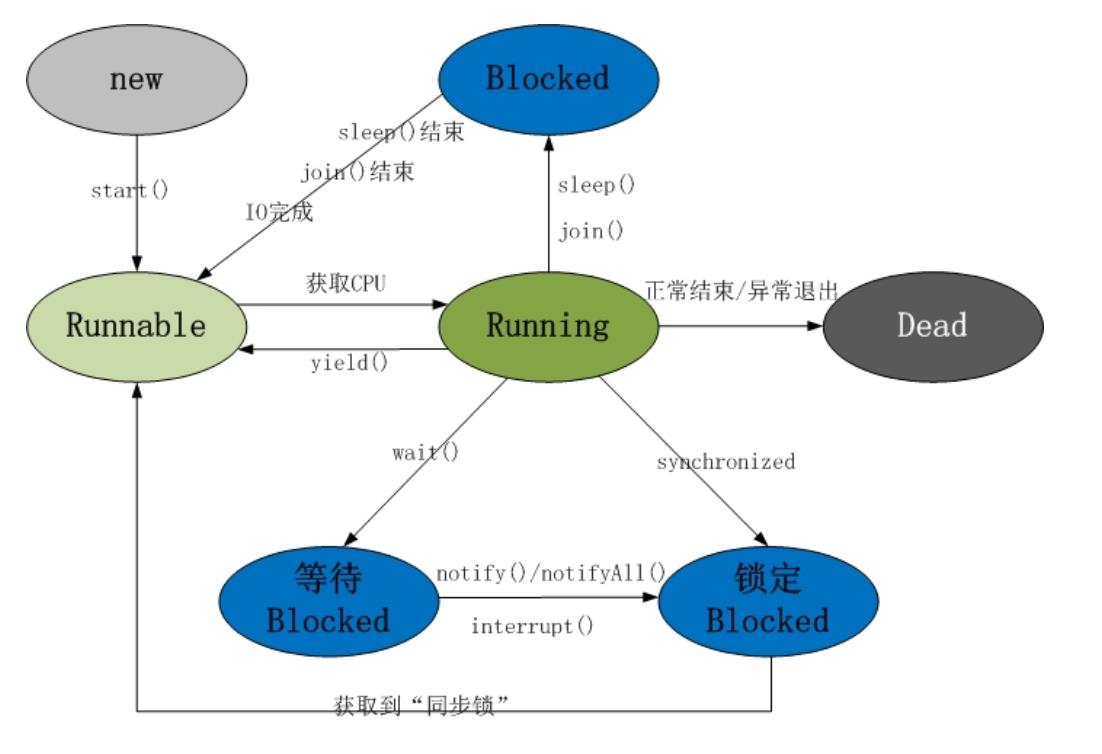# Java总结篇系列：Java多线程（一）Java线程具有五中基本状态

1.等待阻塞：运行状态中的线程执行wait()方法，使本线程进入到等待阻塞状态；

2.同步阻塞 — 线程在获取synchronized同步锁失败(因为锁被其它线程所占用)，它会进入同步阻塞状态；

3.其他阻塞 — 通过调用线程的sleep()或join()或发出了I/O请求时，线程会进入到阻塞状态。当sleep()状态超时、join()等待线程终止或者超时、或者I/O处理完毕时，线程重新转入就绪状态。

Java中线程的创建常见有如三种基本形式

``` 1 class MyThread extends Thread {
2
3     private int i = 0;
4
5     @Override
6     public void run() {
7         for (i = 0; i < 100; i++) {
9         }
10     }
11 }```
``` 1 public class ThreadTest {
2
3     public static void main(String[] args) {
4         for (int i = 0; i < 100; i++) {
6             if (i == 30) {
11             }
12         }
13     }
14 }```

``` 1 class MyRunnable implements Runnable {
2     private int i = 0;
3
4     @Override
5     public void run() {
6         for (i = 0; i < 100; i++) {
8         }
9     }
10 }```
``` 1 public class ThreadTest {
2
3     public static void main(String[] args) {
4         for (int i = 0; i < 100; i++) {
6             if (i == 30) {
7                 Runnable myRunnable = new MyRunnable(); // 创建一个Runnable实现类的对象
12             }
13         }
14     }
15 }```

``` 1 public class ThreadTest {
2
3     public static void main(String[] args) {
4         for (int i = 0; i < 100; i++) {
6             if (i == 30) {
7                 Runnable myRunnable = new MyRunnable();
10             }
11         }
12     }
13 }
14
15 class MyRunnable implements Runnable {
16     private int i = 0;
17
18     @Override
19     public void run() {
20         System.out.println("in MyRunnable run");
21         for (i = 0; i < 100; i++) {
23         }
24     }
25 }
26
28
29     private int i = 0;
30
32         super(runnable);
33     }
34
35     @Override
36     public void run() {
38         for (i = 0; i < 100; i++) {
40         }
41     }
42 }```

`1 Thread thread = new MyThread(myRunnable);`

```1 public interface Runnable {
2
3     public abstract void run();
4
5 }```

``` 1 public class ThreadTest {
2
3     public static void main(String[] args) {
4
5         Callable<Integer> myCallable = new MyCallable();    // 创建MyCallable对象
7
8         for (int i = 0; i < 100; i++) {
10             if (i == 30) {
13             }
14         }
15
16         System.out.println("主线程for循环执行完毕..");
17
18         try {
19             int sum = ft.get();            //取得新创建的新线程中的call()方法返回的结果
20             System.out.println("sum = " + sum);
21         } catch (InterruptedException e) {
22             e.printStackTrace();
23         } catch (ExecutionException e) {
24             e.printStackTrace();
25         }
26
27     }
28 }
29
30
31 class MyCallable implements Callable<Integer> {
32     private int i = 0;
33
34     // 与run()方法不同的是，call()方法具有返回值
35     @Override
36     public Integer call() {
37         int sum = 0;
38         for (; i < 100; i++) {
40             sum += i;
41         }
42         return sum;
43     }
44
45 }```

```1 public class FutureTask<V> implements RunnableFuture<V> {
2
3     //....
4
5 }```
```1 public interface RunnableFuture<V> extends Runnable, Future<V> {
2
3     void run();
4
5 }```

``` 1 public class ThreadTest {
2
3     public static void main(String[] args) {
4
5         MyRunnable myRunnable = new MyRunnable();
7
8         for (int i = 0; i < 100; i++) {
10             if (i == 30) {
12             }
13             if(i == 40){
15             }
16         }
17     }
18 }
19
20 class MyRunnable implements Runnable {
21
22     private boolean stop;
23
24     @Override
25     public void run() {
26         for (int i = 0; i < 100 && !stop; i++) {